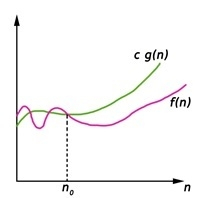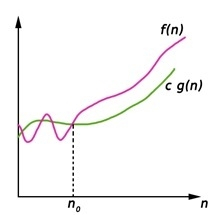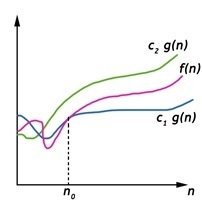# Asymptotic Notations

Analysis of AlgorithmsData StructureAlgorithms

## Asymptotic Notations

Asymptotic notations are used to represent the complexities of algorithms for asymptotic analysis. These notations are mathematical tools to represent the complexities. There are three notations that are commonly used.

## Big Oh Notation

Big-Oh (O) notation gives an upper bound for a function f(n) to within a constant factor.We write f(n) = O(g(n)), If there are positive constantsn0  and c such that, to the right of n0 the f(n) always lies on or below c*g(n).

O(g(n)) = { f(n) : There exist positive constant c and n0 such that 0 ≤ f(n) ≤ c g(n), for all n ≥ n0}

## Big Omega Notation

Big-Omega (Ω) notation gives a lower bound for a function f(n) to within a constant factor.We write f(n) = Ω(g(n)), If there are positive constantsn0  and c such that, to the right of n0 the f(n) always lies on or above c*g(n).

Ω(g(n)) = { f(n) : There exist positive constant c and n0 such that 0 ≤ c g(n) ≤ f(n), for all n ≥ n0}

## Big Theta Notation

Big-Theta(Θ) notation gives bound for a function f(n) to within a constant factor.We write f(n) = Θ(g(n)), If there are positive constantsn0  and c1 and c2 such that, to the right of n0 the f(n) always lies between c1*g(n) and c2*g(n) inclusive.

Θ(g(n)) = {f(n) : There exist positive constant c1, c2 and n0 such that 0 ≤ c1 g(n) ≤ f(n) ≤ c2 g(n), for all n ≥ n0}